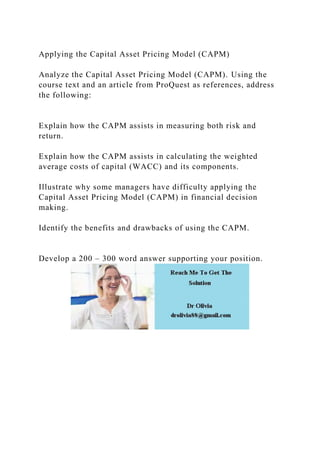Successfully reported this slideshow.

# Applying the Capital Asset Pricing Model (CAPM)Analyze the Capit.docx×

# Applying the Capital Asset Pricing Model (CAPM)Analyze the Capit.docx

Applying the Capital Asset Pricing Model (CAPM)

Analyze the Capital Asset Pricing Model (CAPM). Using the course text and an article from ProQuest as references, address the following:

Explain how the CAPM assists in measuring both risk and return.

Explain how the CAPM assists in calculating the weighted average costs of capital (WACC) and its components.

Illustrate why some managers have difficulty applying the Capital Asset Pricing Model (CAPM) in financial decision making.

Identify the benefits and drawbacks of using the CAPM.

.

Applying the Capital Asset Pricing Model (CAPM)

Analyze the Capital Asset Pricing Model (CAPM). Using the course text and an article from ProQuest as references, address the following:

Explain how the CAPM assists in measuring both risk and return.

Explain how the CAPM assists in calculating the weighted average costs of capital (WACC) and its components.

Illustrate why some managers have difficulty applying the Capital Asset Pricing Model (CAPM) in financial decision making.

Identify the benefits and drawbacks of using the CAPM.